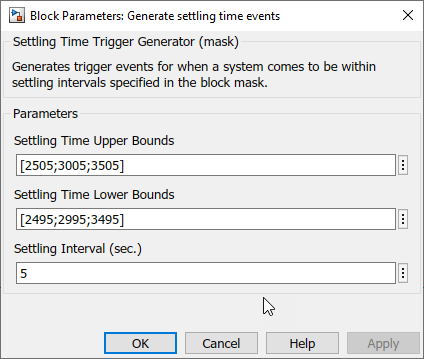# Compute Operating Point Snapshots at Triggered Events

This example shows how to generate operating points using triggered simulation snapshots.

### Open Model

The model for this example is a speed control system.

Open the model.

```mdl = "scdspeedtrigger"; open_system(mdl) ```The Reference Steps block generates a reference signal that steps through three steady-state speed conditions: 2500, 3000, and 3500 rpm. In this example, you find operating points at each of these conditions by taking operating point snapshots.

### Configure Settling Time Events

Since the exact time that a system reaches a steady-state condition is not always known, you can configure your model to detect when a steady-state condition occurs and generate corresponding trigger events.

For this example, the Generate settling time events subsystem detects when the speed signal near a steady-state settling point. The block generates a trigger event when the input signal is within a specified region near the settling point for a minimum amount of time.

For this example, you define regions near the three steady-state speed values. Open the block and specify the upper and lower bounds for these ranges to be 5 rpm above and below the steady-state speed values. To do so, set the Settling Time Upper Bounds and Settling Time Lower Bounds parameters.

Also, specify a minimum settling interval of 5 seconds using the Settling Interval parameter.Within the Generate settling time events subsystem:

• When the input signal is within the specified upper and lower bounds, the Interval Test Dynamic block outputs a `true` signal.

• The Interval Test Dynamic block output changing from `false` to `true` triggers a latching mechanism to track how long the signal is `true`.

• When the signal is `true` for a specified interval time, the latching mechanism outputs a `true` signal.

• When the outputs of the Interval Test Dynamic block and the latching mechanism are both `true`, the output trigger signal is set to `true`.The trigger signal from the Generate settling time events subsystem connects to a Trigger-Based Operating Point Snapshot block. You can configure this block to take operating point snapshots on the rising or falling edge of a trigger signal. For this example, the block uses the rising edge of the trigger signal.

### Find Operating Points

To compute the operating points, use the `findop` function to simulate the model for 60 seconds. This function returns a vector of four operating points: one for each triggered snapshot time and one at a simulation time of 60 seconds.

```op = findop(mdl,60); ```

The first operating point is near the 2500 rpm (261.8 rad/s) settling condition.

```op(1) ```
```ans = Operating point for the Model scdspeedtrigger. (Time-Varying Components Evaluated at time t=10.63) States: ---------- x ________ (1.) scdspeedtrigger/PID Controller/Filter/Cont. Filter/Filter 0 (2.) scdspeedtrigger/PID Controller/Integrator/Continuous/Integrator 10.4701 (3.) scdspeedtrigger/Throttle & Manifold/Intake Manifold/p0 = 0.543 bar 0.51066 (4.) scdspeedtrigger/Vehicle Dynamics/w = T//J w0 = 209 rad//s 261.7988 Inputs: None ---------- ```

The second operating point is near the 3000 rpm (314.16 rad/s) settling condition.

```op(2) ```
```ans = Operating point for the Model scdspeedtrigger. (Time-Varying Components Evaluated at time t=28.3703) States: ---------- x ________ (1.) scdspeedtrigger/PID Controller/Filter/Cont. Filter/Filter 0 (2.) scdspeedtrigger/PID Controller/Integrator/Continuous/Integrator 11.9151 (3.) scdspeedtrigger/Throttle & Manifold/Intake Manifold/p0 = 0.543 bar 0.49012 (4.) scdspeedtrigger/Vehicle Dynamics/w = T//J w0 = 209 rad//s 314.1596 Inputs: None ---------- ```

The third operating point is near the 3500 rpm (366.52 rad/s) settling condition.

```op(3) ```
```ans = Operating point for the Model scdspeedtrigger. (Time-Varying Components Evaluated at time t=48.2688) States: ---------- x _______ (1.) scdspeedtrigger/PID Controller/Filter/Cont. Filter/Filter 0 (2.) scdspeedtrigger/PID Controller/Integrator/Continuous/Integrator 13.3488 (3.) scdspeedtrigger/Throttle & Manifold/Intake Manifold/p0 = 0.543 bar 0.47835 (4.) scdspeedtrigger/Vehicle Dynamics/w = T//J w0 = 209 rad//s 366.52 Inputs: None ---------- ```

For an example that linearizes the speed control model at these operating points, see Linearize at Triggered Simulation Events.

```bdclose(mdl) ```